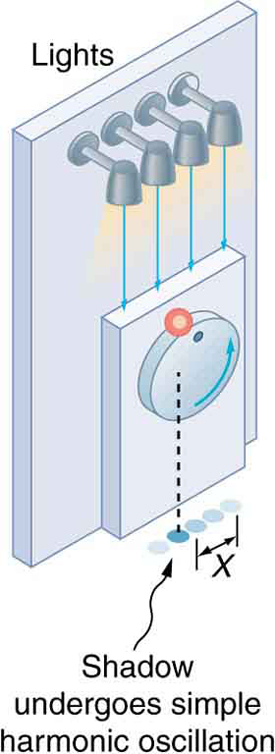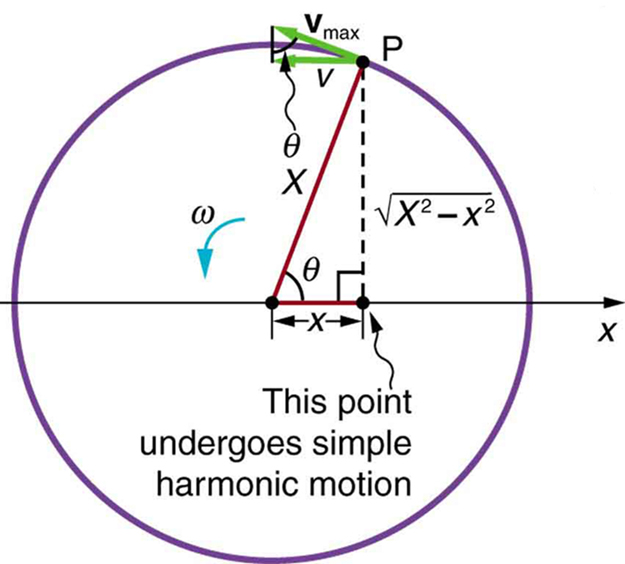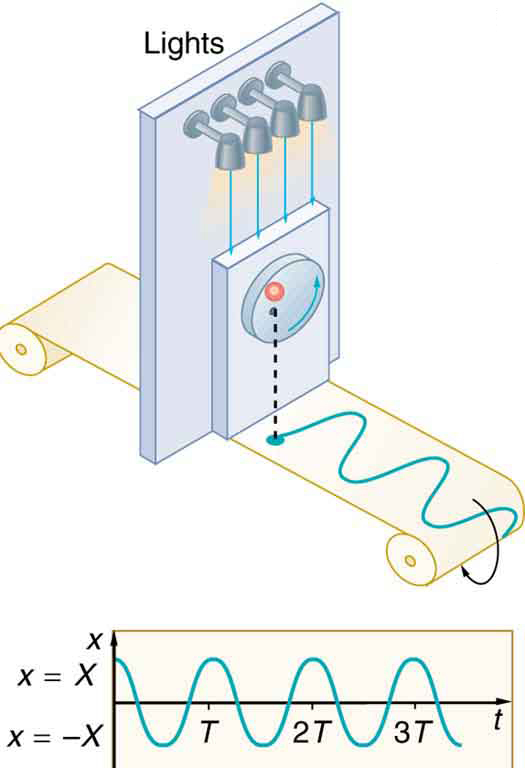# 16.6 Uniform circular motion and simple harmonic motion

 Page 1 / 1
• Compare simple harmonic motion with uniform circular motion.The horses on this merry-go-round exhibit uniform circular motion. (credit: Wonderlane, Flickr)

There is an easy way to produce simple harmonic motion by using uniform circular motion. [link] shows one way of using this method. A ball is attached to a uniformly rotating vertical turntable, and its shadow is projected on the floor as shown. The shadow undergoes simple harmonic motion. Hooke’s law usually describes uniform circular motions ( $\omega$ constant) rather than systems that have large visible displacements. So observing the projection of uniform circular motion, as in [link] , is often easier than observing a precise large-scale simple harmonic oscillator. If studied in sufficient depth, simple harmonic motion produced in this manner can give considerable insight into many aspects of oscillations and waves and is very useful mathematically. In our brief treatment, we shall indicate some of the major features of this relationship and how they might be useful.The shadow of a ball rotating at constant angular velocity ω size 12{ω} {} on a turntable goes back and forth in precise simple harmonic motion.

[link] shows the basic relationship between uniform circular motion and simple harmonic motion. The point P travels around the circle at constant angular velocity $\omega$ . The point P is analogous to an object on the merry-go-round. The projection of the position of P onto a fixed axis undergoes simple harmonic motion and is analogous to the shadow of the object. At the time shown in the figure, the projection has position $x$ and moves to the left with velocity $v$ . The velocity of the point P around the circle equals ${\overline{v}}_{\text{max}}$ .The projection of ${\overline{v}}_{\text{max}}$ on the $x$ -axis is the velocity $v$ of the simple harmonic motion along the $x$ -axis.A point P moving on a circular path with a constant angular velocity ω size 12{ω} {} is undergoing uniform circular motion. Its projection on the x-axis undergoes simple harmonic motion. Also shown is the velocity of this point around the circle, v ¯ max size 12{ {overline {v}} rSub { size 8{"max"} } } {} , and its projection, which is v size 12{v} {} . Note that these velocities form a similar triangle to the displacement triangle.

To see that the projection undergoes simple harmonic motion, note that its position $x$ is given by

$x=X\phantom{\rule{0.25em}{0ex}}\text{cos}\phantom{\rule{0.25em}{0ex}}\theta \text{,}$

where $\theta =\omega t$ , $\omega$ is the constant angular velocity, and $X$ is the radius of the circular path. Thus,

$x=X\phantom{\rule{0.25em}{0ex}}\text{cos}\phantom{\rule{0.25em}{0ex}}\omega t.$

The angular velocity $\omega$ is in radians per unit time; in this case $2\pi$ radians is the time for one revolution $T$ . That is, $\omega =2\pi /T$ . Substituting this expression for $\omega$ , we see that the position $x$ is given by:

$x\left(t\right)=\text{cos}\left(\frac{2\pi t}{T}\right).$

This expression is the same one we had for the position of a simple harmonic oscillator in Simple Harmonic Motion: A Special Periodic Motion . If we make a graph of position versus time as in [link] , we see again the wavelike character (typical of simple harmonic motion) of the projection of uniform circular motion onto the $x$ -axis.The position of the projection of uniform circular motion performs simple harmonic motion, as this wavelike graph of x size 12{x} {} versus t size 12{x} {} indicates.

Now let us use [link] to do some further analysis of uniform circular motion as it relates to simple harmonic motion. The triangle formed by the velocities in the figure and the triangle formed by the displacements ( $X,\phantom{\rule{0.25em}{0ex}}x,\phantom{\rule{0.25em}{0ex}}$ and $\sqrt{{X}^{2}-{x}^{2}}$ ) are similar right triangles. Taking ratios of similar sides, we see that

$\frac{v}{{v}_{\text{max}}}=\frac{\sqrt{{X}^{2}-{x}^{2}}}{X}=\sqrt{1-\frac{{x}^{2}}{{X}^{2}}}.$

We can solve this equation for the speed $v$ or

$v={v}_{\text{max}}\sqrt{1-\frac{{x}^{2}}{{X}^{2}}}.$

This expression for the speed of a simple harmonic oscillator is exactly the same as the equation obtained from conservation of energy considerations in Energy and the Simple Harmonic Oscillator .You can begin to see that it is possible to get all of the characteristics of simple harmonic motion from an analysis of the projection of uniform circular motion.

Finally, let us consider the period $T$ of the motion of the projection. This period is the time it takes the point P to complete one revolution. That time is the circumference of the circle $2\pi X$ divided by the velocity around the circle, ${v}_{\text{max}}$ . Thus, the period $T$ is

$T=\frac{2\pi X}{{v}_{\text{max}}}.$

We know from conservation of energy considerations that

${v}_{\text{max}}=\sqrt{\frac{k}{m}}X.$

Solving this equation for $X/{v}_{\text{max}}$ gives

$\frac{X}{{v}_{\text{max}}}=\sqrt{\frac{m}{k}}.$

Substituting this expression into the equation for $T$ yields

$T=2\pi \sqrt{\frac{m}{k}}\text{.}$

Thus, the period of the motion is the same as for a simple harmonic oscillator. We have determined the period for any simple harmonic oscillator using the relationship between uniform circular motion and simple harmonic motion.

Some modules occasionally refer to the connection between uniform circular motion and simple harmonic motion. Moreover, if you carry your study of physics and its applications to greater depths, you will find this relationship useful. It can, for example, help to analyze how waves add when they are superimposed.

Identify an object that undergoes uniform circular motion. Describe how you could trace the simple harmonic motion of this object as a wave.

A record player undergoes uniform circular motion. You could attach dowel rod to one point on the outside edge of the turntable and attach a pen to the other end of the dowel. As the record player turns, the pen will move. You can drag a long piece of paper under the pen, capturing its motion as a wave.

## Section summary

A projection of uniform circular motion undergoes simple harmonic oscillation.

## Problems&Exercises

(a)What is the maximum velocity of an 85.0-kg person bouncing on a bathroom scale having a force constant of $1\text{.}\text{50}×{\text{10}}^{6}\phantom{\rule{0.25em}{0ex}}\text{N/m}$ , if the amplitude of the bounce is 0.200 cm? (b)What is the maximum energy stored in the spring?

a). 0.266 m/s

b). 3.00 J

A novelty clock has a 0.0100-kg mass object bouncing on a spring that has a force constant of 1.25 N/m. What is the maximum velocity of the object if the object bounces 3.00 cm above and below its equilibrium position? (b) How many joules of kinetic energy does the object have at its maximum velocity?

At what positions is the speed of a simple harmonic oscillator half its maximum? That is, what values of $x/X$ give $v=±{v}_{\text{max}}/2$ , where $X$ is the amplitude of the motion?

$±\frac{\sqrt{3}}{2}$

A ladybug sits 12.0 cm from the center of a Beatles music album spinning at 33.33 rpm. What is the maximum velocity of its shadow on the wall behind the turntable, if illuminated parallel to the record by the parallel rays of the setting Sun?

how does a model differ from a theory
what is vector quantity
Vector quality have both direction and magnitude, such as Force, displacement, acceleration and etc.
Besmellah
Is the force attractive or repulsive between the hot and neutral lines hung from power poles? Why?
what's electromagnetic induction
electromagnetic induction is a process in which conductor is put in a particular position and magnetic field keeps varying.
Lukman
wow great
Salaudeen
what is mutual induction?
je
mutual induction can be define as the current flowing in one coil that induces a voltage in an adjacent coil.
Johnson
how to undergo polarization
show that a particle moving under the influence of an attractive force mu/y³ towards the axis x. show that if it be projected from the point (0,k) with the component velocities U and V parallel to the axis of x and y, it will not strike the axis of x unless u>v²k² and distance uk²/√u-vk as origin
show that a particle moving under the influence of an attractive force mu/y^3 towards the axis x. show that if it be projected from the point (0,k) with the component velocities U and V parallel to the axis of x and y, it will not strike the axis of x unless u>v^2k^2 and distance uk^2/√u-k as origin
No idea.... Are you even sure this question exist?
Mavis
I can't even understand the question
yes it was an assignment question "^"represent raise to power pls
Gabriel
Gabriel
An engineer builds two simple pendula. Both are suspended from small wires secured to the ceiling of a room. Each pendulum hovers 2 cm above the floor. Pendulum 1 has a bob with a mass of 10kg . Pendulum 2 has a bob with a mass of 100 kg . Describe how the motion of the pendula will differ if the bobs are both displaced by 12º .
no ideas
Augstine
if u at an angle of 12 degrees their period will be same so as their velocity, that means they both move simultaneously since both both hovers at same length meaning they have the same length
Modern cars are made of materials that make them collapsible upon collision. Explain using physics concept (Force and impulse), how these car designs help with the safety of passengers.
calculate the force due to surface tension required to support a column liquid in a capillary tube 5mm. If the capillary tube is dipped into a beaker of water
find the time required for a train Half a Kilometre long to cross a bridge almost kilometre long racing at 100km/h
method of polarization
Ajayi
What is atomic number?
The number of protons in the nucleus of an atom
Deborah
type of thermodynamics
oxygen gas contained in a ccylinder of volume has a temp of 300k and pressure 2.5×10Nm
why the satellite does not drop to the earth explain
what is a matter
Yinka
what is matter
Yinka
what is matter
Yinka
what is a matter
Yinka
I want the nuclear physics conversation
Mohamed
because space is a vacuum and anything outside the earth 🌎 can not come back without an act of force applied to it to leave the vacuum and fall down to the earth with a maximum force length of 30kcm per second
Clara
at t=0second,aparticles moving in x-y plain with aconstant acceleration has avelocity of initial velocity =(3i-2j)m/s and is at the origion.at t=3second the particle's velocity is final velocity=(9i+7j)then how to find the acceleration?
how about the formula like v^2=u^2+2as
Bayuo
a=v-u/t
Doreen
what is physics
Yinka

#### Get Jobilize Job Search Mobile App in your pocket Now!ByBy OpenStaxBy Anonymous UserBy Sheila LopezBy OpenStaxBy Samuel MaddenBy Stephanie RedfernBy OpenStaxBy OpenStaxBy OpenStaxBy Jonathan Long